# ORDINARY DIFFERENTIAL EQUATION

19 Mar 2019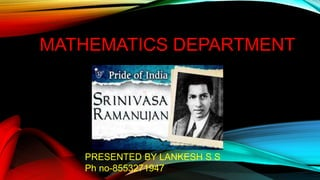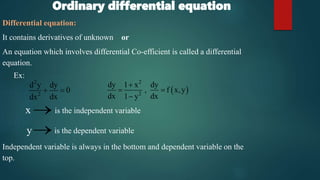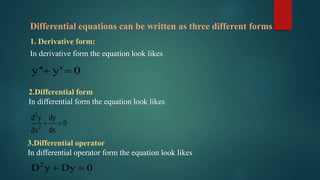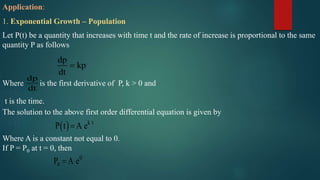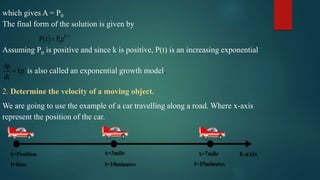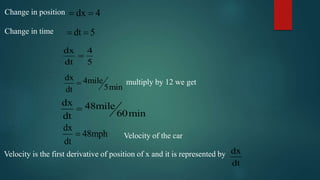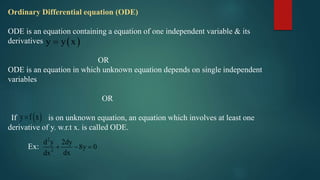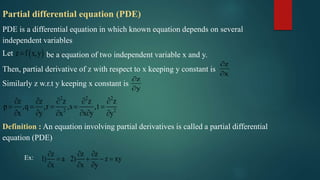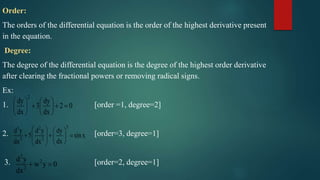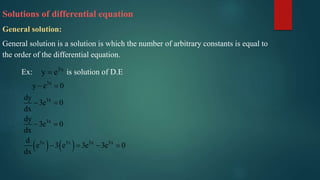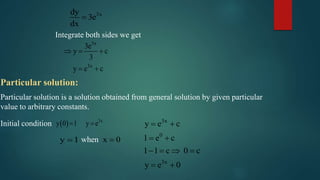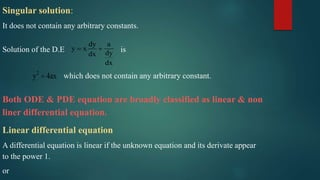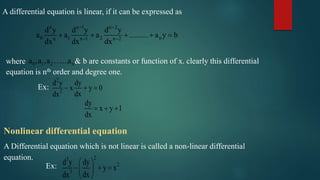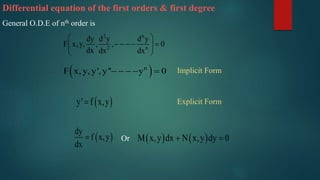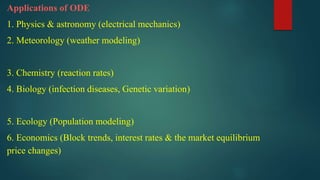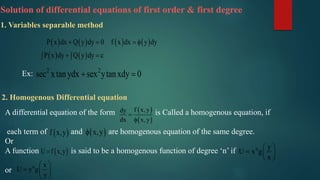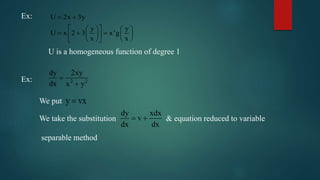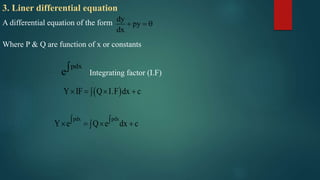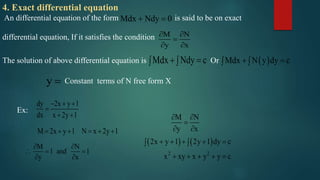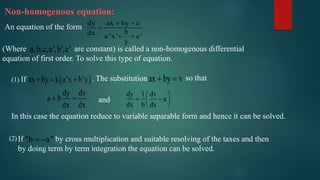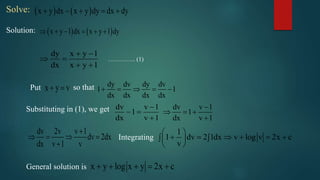1 sur 23

### ORDINARY DIFFERENTIAL EQUATION

• 1. MATHEMATICS DEPARTMENT PRESENTED BY LANKESH S S Ph no-8553271947
• 2. Ordinary differential equation Differential equation: It contains derivatives of unknown or An equation which involves differential Co-efficient is called a differential equation. Independent variable is always in the bottom and dependent variable on the top. 2 2 d y dy 0 dxdx   Ex: x  is the independent variable y is the dependent variable   2 2 dy 1 x dy , f x,y dx dx1 y    
• 3. Differential equations can be written as three different forms 1. Derivative form: In derivative form the equation look likes y'' y' 0  2.Differential form In differential form the equation look likes 2 2 d y dy 0 dxdx   3.Differential operator In differential operator form the equation look likes 2 D y Dy 0 
• 4. Application: 1. Exponential Growth – Population Let P(t) be a quantity that increases with time t and the rate of increase is proportional to the same quantity P as follows Where is the first derivative of P, k > 0 and t is the time. The solution to the above first order differential equation is given by Where A is a constant not equal to 0. If P = P0 at t = 0, then dp kp dt  dp dt   k t P t A e 0 0P A e
• 5. which gives A = P0 The final form of the solution is given by   k t 0P t P e Assuming P0 is positive and since k is positive, P(t) is an increasing exponential. dp kp dt  is also called an exponential growth model. 2. Determine the velocity of a moving object. We are going to use the example of a car travelling along a road. Where x-axis represent the position of the car.
• 6. Change in position Change in time dx 4  dt 5  dx 4 dt 5  dx 4mile 5mindt  multiply by 12 we get dx 48mile 60mindt  Velocity of the car dx 48mph dt  Velocity is the first derivative of position of x and it is represented by dx dt
• 7. Ordinary Differential equation (ODE) ODE is an equation containing a equation of one independent variable & its derivatives OR ODE is an equation in which unknown equation depends on single independent variables OR If is on unknown equation, an equation which involves at least one derivative of y. w.r.t x. is called ODE. Ex:  y y x  y f x 2 2 d y 2dy 8y 0 dxdx   
• 8. Partial differential equation (PDE) PDE is a differential equation in which known equation depends on several independent variables be a equation of two independent variable x and y. Then, partial derivative of z with respect to x keeping y constant is Similarly z w.r.t y keeping x constant is Definition : An equation involving partial derivatives is called a partial differential equation (PDE) Let  z f x,y z x   z y   2 2 2 2 2 z z z z z p ,q ,r ,s ,t x y x yx y                Ex: z z z 1) a 2) z xy x x y          
• 9. Order: The orders of the differential equation is the order of the highest derivative present in the equation. Degree: The degree of the differential equation is the degree of the highest order derivative after clearing the fractional powers or removing radical signs. Ex: 1. [order =1, degree=2] 2. [order=3, degree=1] 3. [order=2, degree=1] 2 dy dy 3 2 0 dx dx               33 2 2 2 d y d y dy 5 sinx dxdx dx              2 2 2 d y w y 0 dx  
• 10. Solutions of differential equation General solution: General solution is a solution is which the number of arbitrary constants is equal to the order of the differential equation. 3x y eEx: is solution of D.E     3x 3x 3x 3x 3x 3x 3x y e 0 dy 3e 0 dx dy 3e 0 dx d e 3 e 3e 3e 0 dx          
• 11. 3xdy 3e dx  Integrate both sides we get 3x 3x 3e y c 3 y e c      Particular solution: Particular solution is a solution obtained from general solution by given particular value to arbitrary constants. Initial condition   3x y 0 1 y e  y 1 x 0when 3x 0 3x y e c 1 e c 1 1 c 0 c y e 0          
• 12. Singular solution: It does not contain any arbitrary constants. Solution of the D.E is dy a y x dydx dx   2 y 4ax which does not contain any arbitrary constant. Both ODE & PDE equation are broadly classified as linear & non liner differential equation. Linear differential equation A differential equation is linear if the unknown equation and its derivate appear to the power 1. or
• 13. A differential equation is linear, if it can be expressed as n n 1 n 2 0 1 2 nn n 1 n 2 d y d y d y a a a .......... a y b dx dx dx          where 0 1 2 n..a ,a ,a ..a & b are constants or function of x. clearly this differential equation is nth order and degree one. Ex: 2 2 d y dy x y 0 dxdx    dy x y 1 dx    Nonlinear differential equation A Differential equation which is not linear is called a non-linear differential equation. Ex: 23 2 3 d y dy y x dxdx        
• 14. Differential equation of the first orders & first degree General O.D.E of nth order is 2 n 2 n dy d y d y F x,y, , , 0 dx dx dx            n F x, y, y', y'' y 0      y' f x,y Implicit Form Explicit Form   dy f x,y dx  Or    M x,y dx N x,y dy 0 
• 15. Applications of ODE 1. Physics & astronomy (electrical mechanics) 2. Meteorology (weather modeling) 3. Chemistry (reaction rates) 4. Biology (infection diseases, Genetic variation) 5. Ecology (Population modeling) 6. Economics (Block trends, interest rates & the market equilibrium price changes)
• 16. Solution of differential equations of first order & first degree 1. Variables separable method             P x dx Q y dy 0 f x dx y dy P x dy Q y dy c        Ex: 2 2 sec xtanydx sex ytanxdy 0  2. Homogenous Differential equation A differential equation of the form is Called a homogenous equation, if each term of and are homogenous equation of the same degree. Or A function is said to be a homogenous function of degree ‘n’ if or     f x,ydy dx x,y    f x,y  x,y  U f x,y n y U x g x        n x U y g y       
• 17. Ex: U 2x 3y y y U x 2 3 x'g x x                    U is a homogeneous function of degree 1 2 2 dy 2xy dx x y   Ex: We put y vx We take the substitution & equation reduced to variable separable method dy xdx v dx dx  
• 18. 3. Liner differential equation A differential equation of the form dy py dx    Where P & Q are function of x or constants pdx e Integrating factor (I.F)   pdx pdx Y IF Q I.F dx c Y e Q e dx c         
• 19. 4. Exact differential equation An differential equation of the form is said to be on exact differential equation, If it satisfies the condition Mdx Ndy 0  M N y x      The solution of above differential equation is OrMdx Ndy c    Mdx N y dy c   y  Constant terms of N free form X Ex: dy 2x y 1 dx x 2y 1       M 2x y 1 N x 2y 1      M N 1 and 1 y x            2 2 M N y x 2x y 1 2y 1 dy c x xy x y y c                
• 20. Non-homogenous equation: An equation of the form dy ax by c bdx a 'x ' c' y      (Where are constant) is called a non-homogenous differential equation of first order. To solve this type of equation. a,b,c,a',b',c' (1) If  ax by k a'x b'y   , The substitutionax by v  so that dy dv a b dx dx   In this case the equation reduce to variable separable form and hence it can be solved. dy 1 dv a dx b dx        and (2) If by cross multiplication and suitable resolving of the taxes and then by doing term by term integration the equation can be solved. "b a" 
• 21. Solve:    x y dx x y dy dx dy     Solution:    x y 1 dx x y 1 dy      dy x y 1 dx x y 1       ………….. (1) x y v  dy dv dy dv 1 1 dx dx dx dx     Put so that Substituting in (1), we get dv v 1 1 dx v 1     dv v 1 1 dx v 1      dv 2v v 1 dv 2dx dx v 1 v       1 1 dv 2 1dx v log v 2x c v             Integrating x y log x y 2x c    General solution is